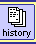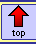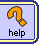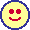## Gauche で bank-account

Gauche には STklos に影響を受けたオブジェクトシステムが標準で搭載されています。

ためしに「CLOS で bank-account」を Gauche 向けに焼き直してみました。いろいろと細かいところは違うようですが、ざっとマニュアルに目を通した感じでは、ほぼ同じ考え方で使えそうです。なお、CLOS ではパラメータ数が異なる同名のメソッドを総称関数に登録できませんでしたが、Gauche では可能なようです。このように Smalltalk ライクにスロット名、ゲッター名、セッター名を揃えてしまう命名法は、あるいは Schemer の不評を買うかもしれませんが、個人的にはイメージ通り、CLOS 版とはひと味違うポリモーフィックな扱いやすいオブジェクトができたので悦に入っています。

STklos にはメタクラスはあるのですが、メタクラスの継承構造とクラスの継承構造を一致させる機構がないので、クラスをオブジェクトと見立てた「お手軽版」プロトタイプベースぽい bank-account（Smalltalk の例Ruby の例）には、それほど“お手軽”感はなさそうです。
• 実際に試してみると、Smalltalk 、Ruby では自動的に定義されるメタクラス(厳密には、Ruby は Smaltalk と違い、クラスメソッド定義時に特異クラスとしてメタクラスが定義される)をいちいち定義しなければいけないので、単にクラスでしていたことをメタクラスを使ってしているだけ…というより、クラスベースでしていることをさらに手数をかけてやっているだけという結果に終わりました。
• 当初書いていた、EQL スペシャライザの有無と、この手法でのプロトタイプベース・プログラミングの可不可は関係ありませんでした(^_^;)。
--sumim

なるほど、屋上屋を架してますか(^_^;)。ですが、クロージャだと委譲機構は自前で用意しないといけないんですよね？ 下の例でいうところの のインスタンスの deposit (今思えば、deposit! とすべきですね…)に対する振る舞いとか。ああ…、これはマクロでなんとかなるわけですね。よい練習になりそうなので、クロージャ版の bank-account をちょっと考えてみます。ありがとうございます。--sumim

…の前に、YASOS を学んでみます。--sumim

```(define-class <bank-account> ()
((dollars :accessor dollars
:init-value 0
:init-keyword :dollars)))

(define-method dollars ((self <bank-account>) x)
(set! (dollars self) x))

(define-method deposit ((self <bank-account>) x)
(dollars self (+ (dollars self) x)))

(define-method withdraw ((self <bank-account>) x)
(dollars self (max 0 (- (dollars self) x))))

(define my-account (make <bank-account> :dollars 200))

(dollars my-account)
==> 200
(deposit my-account 50)
(dollars my-account)
==> 250
(withdraw my-account 100)
(dollars my-account)
==> 150
(withdraw my-account 200)
(dollars my-account)
==> 0

(define-class <stock-account> (<bank-account>)
((num-shares :accessor num-shares
:init-value 0
:init-keyword :num-shares)
(price-per-share  :accessor price-per-share
:init-value 30
:init-keyword :price-per-share)))

(define-method num-shares ((self <stock-account>) x)
(set! (num-shares self) x))

(define-method price-per-share ((self <stock-account>) x)
(set! (price-per-share self) x))

(define-method dollars ((self <stock-account>) x)
(num-shares self (/ x (price-per-share self))))

(define-method dollars ((self <stock-account>))
(* (num-shares self) (price-per-share self)))

(define my-stock (make <stock-account> :num-shares 10))

(dollars my-stock)
==> 300
(dollars my-stock 600)
(dollars my-stock)
==> 600
(deposit my-stock 60)
(dollars my-stock)
==> 660
(num-shares my-stock)
==> 22
(withdraw my-stock 120)
==> 540
(num-shares my-stock)
==> 18```

クラスをオブジェクトにした bank-account を書いてみましたが、上にあるようにクラス−インスタンスでやっていたことを、メタクラス−クラスにシフトしてやっているだけという結果になりました。
```(define-class <bank-account-meta> (<class>)
((dollars :init-value 0)))
(define-class bank-account () () :metaclass <bank-account-meta>)

(define-method dollars ((self <bank-account-meta>))
(slot-ref self 'dollars))

(define-method dollars ((self <bank-account-meta>) x)
(slot-set! self 'dollars x))

(define-method deposit ((self <bank-account-meta>) x)
(dollars self (+ (dollars self) x)))

(define-method withdraw ((self <bank-account-meta>) x)
(dollars self (max 0 (- (dollars self) x))))

(dollars bank-account 200)
(dollars bank-account)
==> 200
(deposit bank-account 50)
(dollars bank-account)
==> 250
(withdraw bank-account 100)
(dollars bank-account)
==> 150
(withdraw bank-account 200)
(dollars bank-account)
==> 0

(define-class <my-account-meta> (<bank-account-meta>) ())
(define-class my-account () () :metaclass <my-account-meta>)

(dollars my-account 500)
(dollars my-account)
==> 500
(dollars bank-account)
==> 0

(define-class <stock-account-meta> (<bank-account-meta>)
((num-shares :init-value 0) (price-per-share :init-value 30)))
(define-class stock-account () () :metaclass <stock-account-meta>)

(define-method num-shares ((self <stock-account-meta>))
(slot-ref self 'num-shares))

(define-method num-shares ((self <stock-account-meta>) x)
(slot-set! self 'num-shares x))

(define-method price-per-share ((self <stock-account-meta>))
(slot-ref self 'price-per-share))

(define-method price-per-share ((self <stock-account-meta>) x)
(set! (price-per-share self) x))

(define-method dollars ((self <stock-account-meta>) x)
(num-shares self (/ x (price-per-share self))))

(define-method dollars ((self <stock-account-meta>))
(* (num-shares self) (price-per-share self)))

(num-shares stock-account 10)
(dollars stock-account)
==> 300
(dollars stock-account 150)
(dollars stock-account)
==> 150
(num-shares stock-account)
==> 5

(define-class <my-stock-meta> (<stock-account-meta>) ())
(define-class my-stock () () :metaclass <my-stock-meta>)

(dollars my-stock 600)
(dollars my-stock)
==> 600
(num-shares my-stock)
==> 20
(num-shares stock-account)
==> 5
(deposit my-stock 60)
(dollars my-stock)
==> 660
(num-shares my-stock)
==> 22
(withdraw my-stock 120)
(dollars my-stock)
==> 540
(num-shares my-stock)
==> 18```

Scheme 的には、ゲッターは dollars-ref 、セッターは dollars-set! とするのがよろしそうですね。--sumim

このページを編集 (6968 bytes)以下の 3 ページから参照されています。